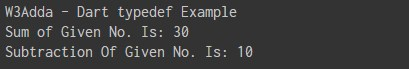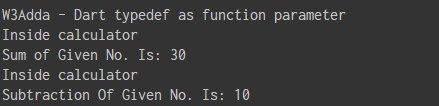Dart Typedef

In this tutorial you will learn about the Dart Typedef and its application with practical example.

Dart Typedef

In Dart, typedef is used to create an alias for function type that you can use as type annotations for declaring variables and return types of that function type. After creating an alias of function type; we can use it as type annotations in variable declaration or in function return type. A typedef holds type information when a function type is assigned to a variable.

Defining a typedef

A typedef keyword can be used to create an alias for function prototype that will comparable with the actual functions. A function prototype specifies function’s parameters (including their types).

Syntax:-

Example:-

Let’s create an alias ManyOperation for a function signature that will take two input parameters of the type integer.

Assigning typedef Variable

A typedef variable can be assigned any function having the same signature as typedef declaration.

Syntax:-

Example:-

Now, lets define some functions with the same function signature as that of the ManyOperation typedef.

Invoking Function with typedef

Now, the typedef variable can be used to invoke functions with same function signature.

Syntax:-

Example:-

The oper variable can be used to refer any method which takes two integer parameters. The typedefs can switch function references in runtime.

Complete Program In Action

Example:-

Output:-Typedefs as Parameter

Lets add one more method to the above program Calculator(int n1,int n2, ManyOperation oper) which accepts two integer numbers (n1 and n2) and one typedef ManyOperation oper as its parameter.

Example:-

Output:-In this tutorial we have learn about the Dart Typedef and its application with practical example. I hope you will like this tutorial.